# Acids and Bases Electrolytes Substance that conducts electricity

• Slides: 24Acids and Bases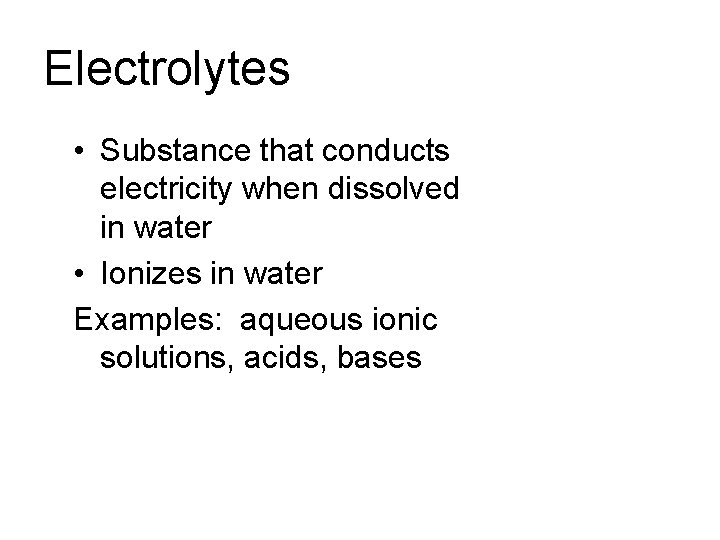Electrolytes • Substance that conducts electricity when dissolved in water • Ionizes in water Examples: aqueous ionic solutions, acids, basesProperties of Acids • • • Acids have a p. H less than 7. 0 Acids will burn your skin Dilute acids have a sour taste Strong acids are good conductors of electricity Acids react with bases to form water and a salt (neutralization reaction) • Acids react with certain metals to produce hydrogen gas – Metals above H 2 will react with acids to produce H 2(g)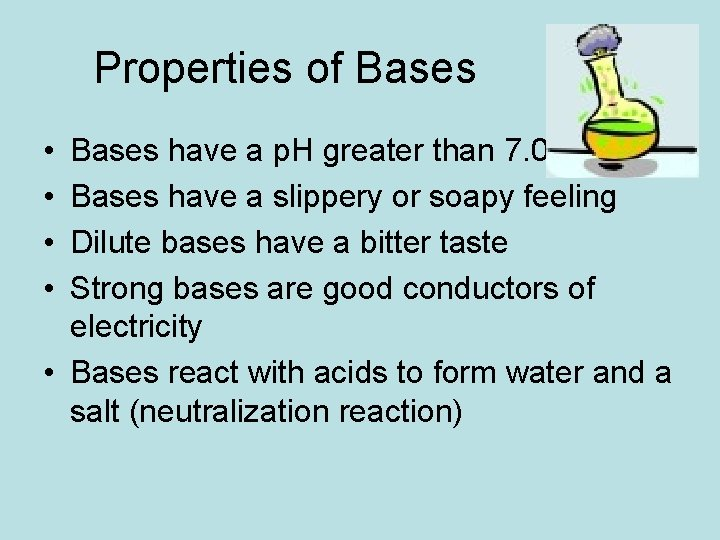Properties of Bases • • Bases have a p. H greater than 7. 0 Bases have a slippery or soapy feeling Dilute bases have a bitter taste Strong bases are good conductors of electricity • Bases react with acids to form water and a salt (neutralization reaction)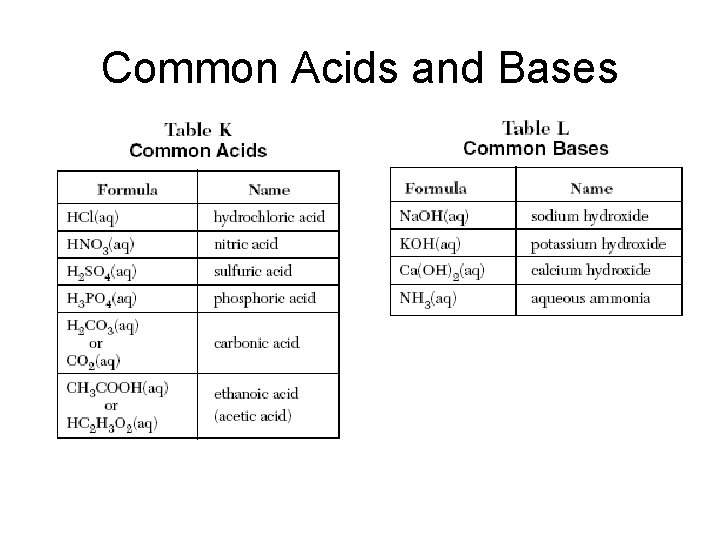Common Acids and Basesp. H Scale • • Scale ranges from 0 -14 Acids = p. H less than 7 Bases = p. H greater than 7 Neutral = p. H = 7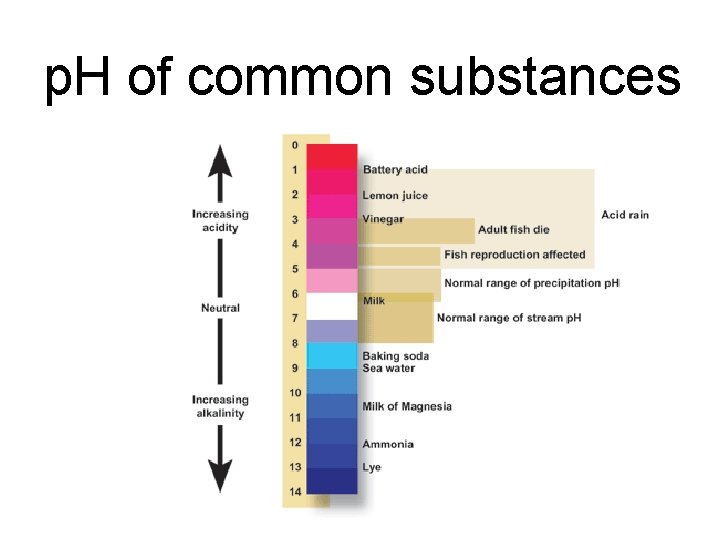p. H of common substances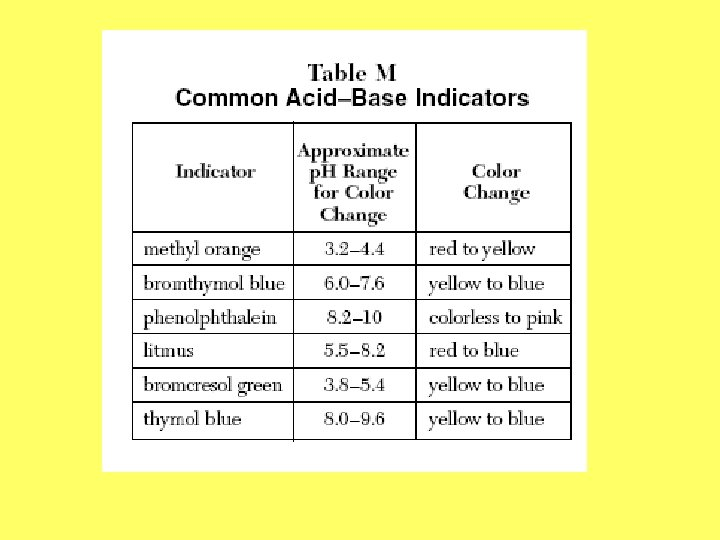Indicators • Common indicators are listed in Table M Examples: 1. What color will thymol blue be in a solution with a p. H of 6. 5? 2. What color will methyl orange turn if a solution has a p. H of 7. 0? 3. What color will phenolphthalein turn in an acid?Arrhenius Theory • Definition of acids and bases • Acids – substances whose water solution contain hydrogen ions (H+), ionize to produce H+ • Bases – substances whose water solutions contain hydroxide ions (OH-), ionize to produce OH • Properties of acids and bases are due to an excess of H+ or OH- ions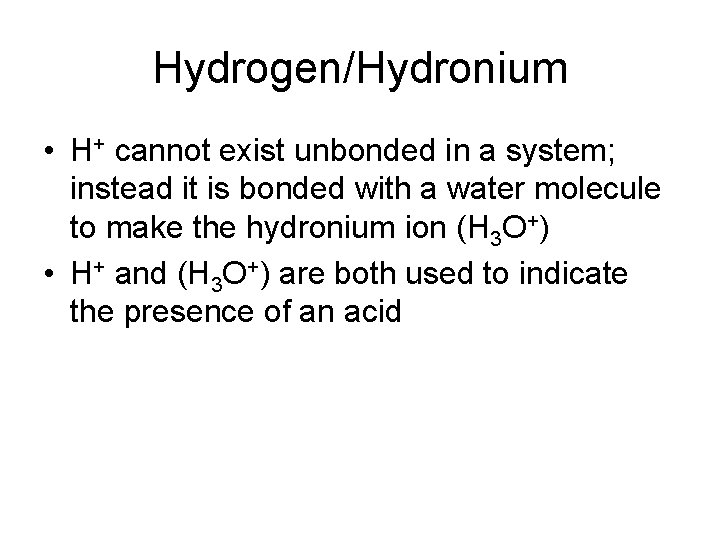Hydrogen/Hydronium • H+ cannot exist unbonded in a system; instead it is bonded with a water molecule to make the hydronium ion (H 3 O+) • H+ and (H 3 O+) are both used to indicate the presence of an acid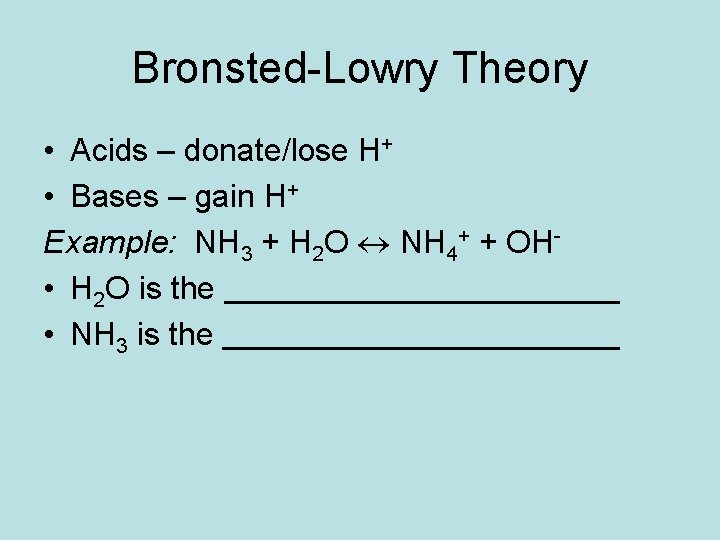Bronsted-Lowry Theory • Acids – donate/lose H+ • Bases – gain H+ Example: NH 3 + H 2 O NH 4+ + OH • H 2 O is the • NH 3 is theStrength of Acids/Bases • Strength is proportional to the degree to which it ionizes in solution • Greater dissociation (more ions), stronger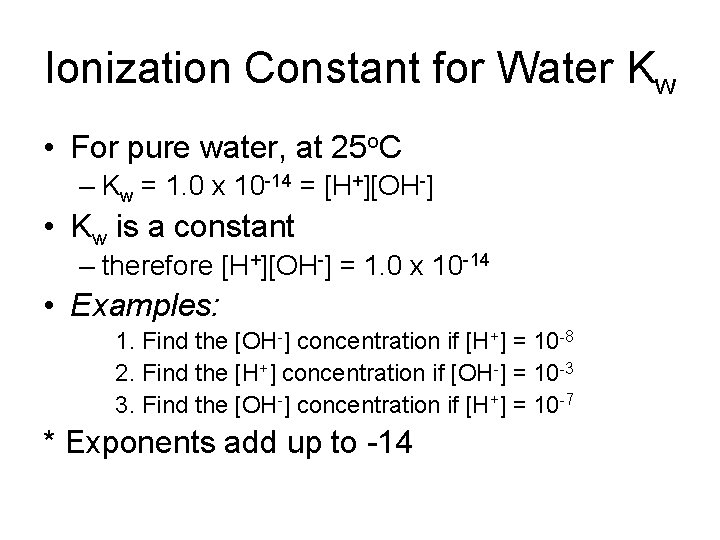Ionization Constant for Water Kw • For pure water, at 25 o. C – Kw = 1. 0 x 10 -14 = [H+][OH-] • Kw is a constant – therefore [H+][OH-] = 1. 0 x 10 -14 • Examples: 1. Find the [OH-] concentration if [H+] = 10 -8 2. Find the [H+] concentration if [OH-] = 10 -3 3. Find the [OH-] concentration if [H+] = 10 -7 * Exponents add up to -14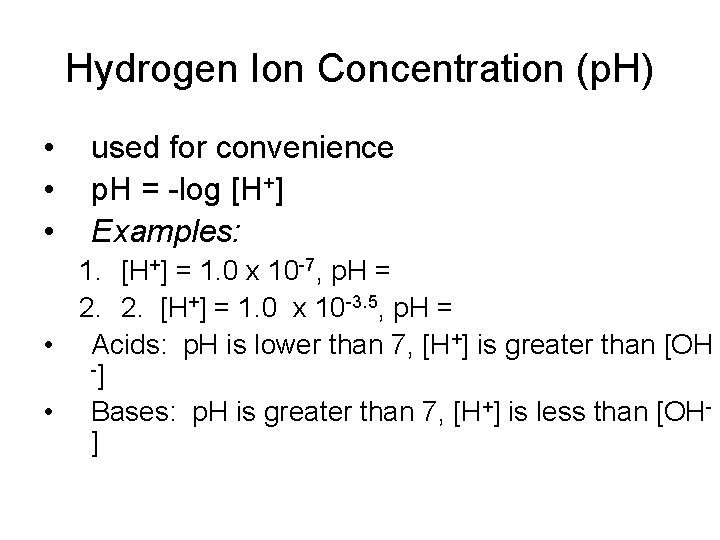Hydrogen Ion Concentration (p. H) • • • used for convenience p. H = -log [H+] Examples: 1. [H+] = 1. 0 x 10 -7, p. H = 2. 2. [H+] = 1. 0 x 10 -3. 5, p. H = • Acids: p. H is lower than 7, [H+] is greater than [OH -] • Bases: p. H is greater than 7, [H+] is less than [OH]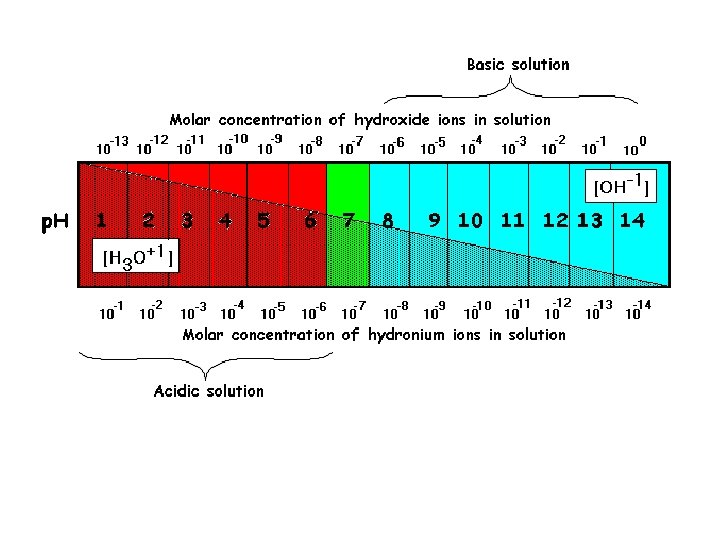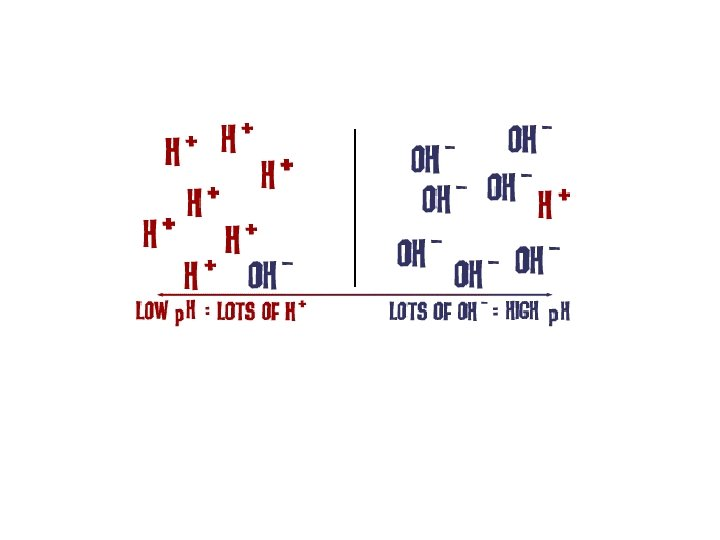Hydroxide Ion Concentration (p. OH) • • p. OH = -log [OH-] Since [H+][OH-] = 1. 0 x 10 -14 p. H + p. OH = 14 Examples: 1. Find the p. OH of a solution with a p. H = 8. Is this solution acidic or basic? 2. Find the p. H of a solution with a p. OH = 12. Is this solution acidic or basic?Concentration and p. H • A change of 1 in p. H is a tenfold increase in acid or base strength – A p. H of 4 is 10 times more acidic than a p. H of 5 – A p. H of 12 is 100 times more basic than a p. H of 10Mono/Di/Triprotic • Monoprotic acids – produce a single hydrogen ion – Examples: HCl, HBr • Diprotic aicds – produce two hydrogen ions – Examples: H 2 SO 4, H 2 S • Triprotic acids – produce three hydrogen ions – Examples: H 3 PO 4Neutralization Reactions • An acid and a base react together to form water and an ionic salt Examples: 1. HCl + Na. OH H 2 O + Na. Cl 2. H 2 SO 4 + Ca(OH)2 2 H 2 O + Ca. SO 4 3. HNO 3 + Ca(OH)2Acid-Base Titrations • Process of adding a measured volume of an acid (or a base) of known molarity to a base (or an acid) of unknown molarity until neutralization occurs – Standard Solution – acid or base of known molarity – End Point – point of neutralization • Unknown molarity is calculated using the titration formula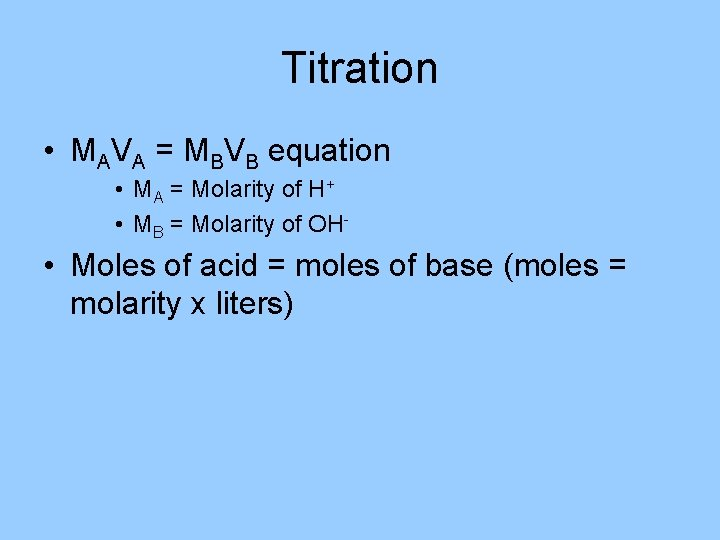Titration • MAVA = MBVB equation • MA = Molarity of H+ • MB = Molarity of OH- • Moles of acid = moles of base (moles = molarity x liters)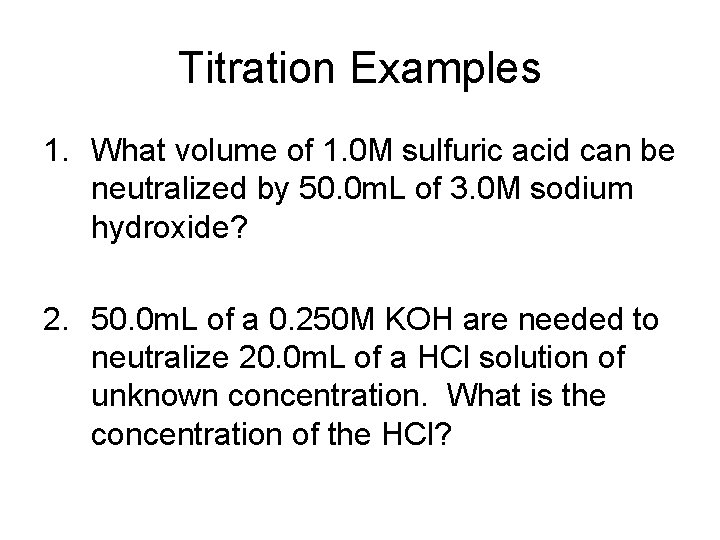Titration Examples 1. What volume of 1. 0 M sulfuric acid can be neutralized by 50. 0 m. L of 3. 0 M sodium hydroxide? 2. 50. 0 m. L of a 0. 250 M KOH are needed to neutralize 20. 0 m. L of a HCl solution of unknown concentration. What is the concentration of the HCl?